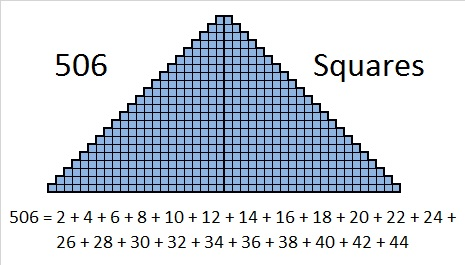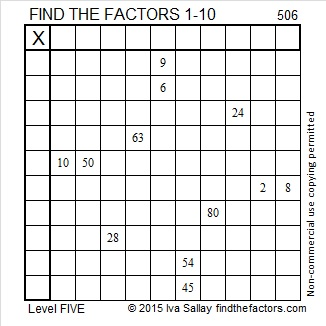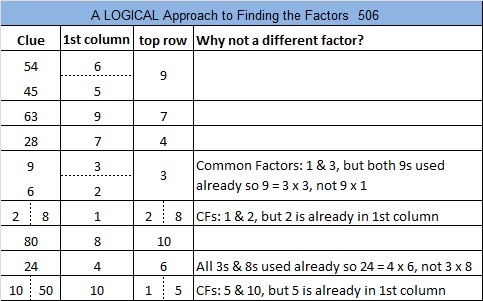# 506 and Level 5

506 is divisible by 11 because 5 + 6 – 0 = 11, and 11 obviously is divisible by 11.

506 is the 11th square pyramidal number because it is the sum of the first eleven square numbers.

Thus 1 + 4 + 9 + 16 + 25 + 36 + 49 + 64 + 81 + 100 + 121 = 506.That was predictable because 506 = (11 x 12 x 23)/6 and 12 = 11 + 1 and 23 = 2(11) + 1.

Since 506 = 22 x 23, it is the sum of the first 22 even numbers which also happens to be exactly two times the 22nd triangular number, 253.Now here’s a Level 5 puzzle for you to try:Print the puzzles or type the solution on this excel file: 10 Factors 2015-05-25

—————————————————————————————————

• 506 is a composite number.
• Prime factorization: 506 = 2 x 11 x 23
• The exponents in the prime factorization are 1, 1, and 1. Adding one to each and multiplying we get (1 + 1)(1 + 1)(1 + 1) = 2 x 2 x 2 = 8. Therefore 506 has exactly 8 factors.
• Factors of 506: 1, 2, 11, 22, 23, 46, 253, 506
• Factor pairs: 506 = 1 x 506, 2 x 253, 11 x 46, or 22 x 33
• 506 has no square factors that allow its square root to be simplified. √506 ≈ 22.49444—————————————————————————————————This site uses Akismet to reduce spam. Learn how your comment data is processed.